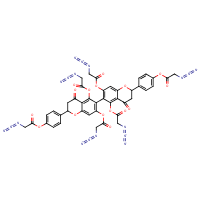Structure InformationCompound Identification

SMILES

[N-]=[N+]=NCC(=O)OC1=CC=C(C=C1)C1CC(=O)C2=C(OC(=O)CN=[N+]=[N-])C(=C(OC(=O)CN=[N+]=[N-])C=C2O1)C1=C(OC(=O)CN=[N+]=[N-])C=C2OC(CC(=O)C2=C1OC(=O)CN=[N+]=[N-])C1=CC=C(OC(=O)CN=[N+]=[N-])C=C1

InChIKey

InChIKey=AGZRDEJMQAMBER-UHFFFAOYSA-N

Formula

C42H28N18O16

Mass

1040.796

### Export to:

Taxonomic Classification

Taxonomy Tree

Kingdom

Organic compounds

Superclass

Organic acids and derivatives

Class

Carboxylic acids and derivatives

Subclass

Hexacarboxylic acids and derivatives

Intermediate Tree Nodes

Not available

Direct Parent

Hexacarboxylic acids and derivatives

Alternative Parents

Molecular Framework

Aromatic heteropolycyclic compounds

Substituents

Hexacarboxylic acid or derivatives - Flavanone - Flavan - Alpha-amino acid ester - Chromone - Alpha-amino acid or derivatives - Chromane - Benzopyran - Phenol ester - 1-benzopyran - Phenoxy compound - Aryl alkyl ketone - Aryl ketone - Alkyl aryl ether - Benzenoid - Monocyclic benzene moiety - Azo compound - Azo imide - Carboxylic acid ester - Ketone - Ether - Oxacycle - Organoheterocyclic compound - Hydrocarbon derivative - Organooxygen compound - Carbonyl group - Organic oxide - Organic oxygen compound - Organonitrogen compound - Organic zwitterion - Organic nitrogen compound - Organic salt - Aromatic heteropolycyclic compound

Description

This compound belongs to the class of organic compounds known as hexacarboxylic acids and derivatives. These are carboxylic acids containing exactly six carboxyl groups.

External Descriptors

Not available